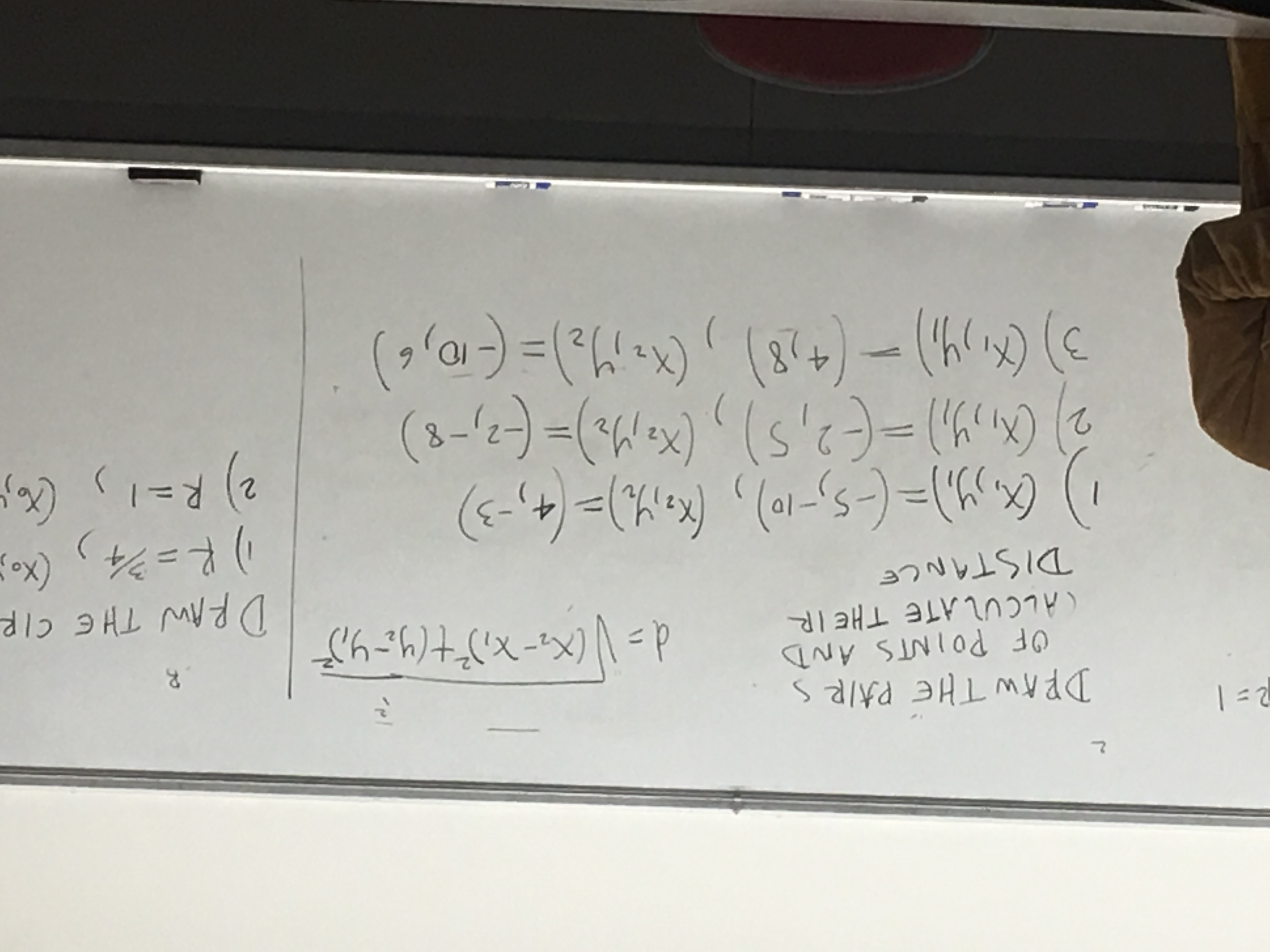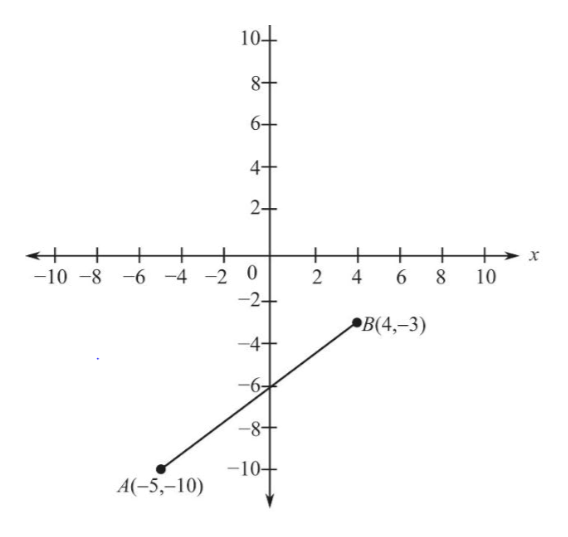# DPAW THE PA/RS2OF POINTS AND(ALCULATE THE IRXx- Xt(M=,D THE CIPDRAW THE CIR(X.DISTANCE2) R=1(XM22-8411

Question
25 views

What would be the easiest ways to solve these problems & graph them?help_outlineImage TranscriptioncloseDPAW THE PA/RS 2 OF POINTS AND (ALCULATE THE IR Xx- Xt(M=, D THE CIP DRAW THE CIR (X. DISTANCE 2) R=1 (XM2 2 -8 4 11 fullscreen
check_circle

star
star
star
star
star
1 Rating
Step 1

According to the given question, it is supposed to calculate the distance between two points.

For that, use the formula:

Step 2

In first part (1) the points are A (-5, -10) and B (4, -3)

So, distance between the points A and B is calculated using the above formula:

Step 3

Graph of the above pair poi...help_outlineImage Transcriptionclose10+ 8+ 6+ 4+ 2+ + -10-8-6 -4-2 0 + 6 2 4 x 8 10 -2+ В(4,-3) 4 10 A(-5,-10) OC fullscreen

### Want to see the full answer?

See Solution

#### Want to see this answer and more?

Solutions are written by subject experts who are available 24/7. Questions are typically answered within 1 hour.*

See Solution
*Response times may vary by subject and question.
Tagged in

### Other